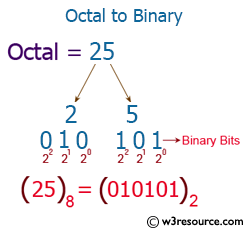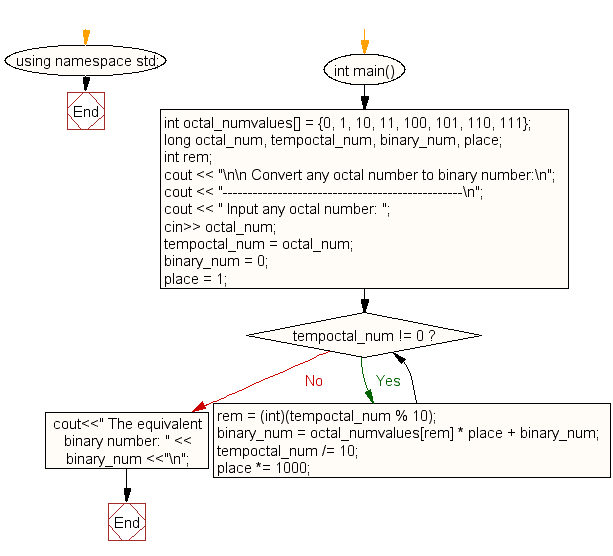﻿ C++ Exercises: Convert a octal number to binary number - w3resource

# C++ Exercises: Convert a octal number to binary number

## C++ For Loop: Exercise-77 with Solution

Write a C++ program to convert an octal number to a binary number.

Pictorial Presentation:Sample Solution:-

C++ Code :

``````#include <iostream>
#include <math.h>
using namespace std;

int main()
{
int octal_numvalues[] = {0, 1, 10, 11, 100, 101, 110, 111};
long octal_num, tempoctal_num, binary_num, place;
int rem;
cout << "\n\n Convert any octal number to binary number:\n";
cout << "------------------------------------------------\n";
cout << " Input any octal number: ";
cin>> octal_num;
tempoctal_num = octal_num;
binary_num = 0;
place = 1;
while (tempoctal_num != 0)
{
rem = (int)(tempoctal_num % 10);
binary_num = octal_numvalues[rem] * place + binary_num;
tempoctal_num /= 10;
place *= 1000;
}
cout<<" The equivalent binary number: " << binary_num <<"\n";
}
``````

Sample Output:

``` Convert any octal number to binary number:
------------------------------------------------
Input any octal number: 17
The equivalent binary number: 1111
```

Flowchart:C++ Code Editor: